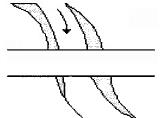Courses

# Test: Surveying- 1

## 25 Questions MCQ Test Civil Engineering SSC JE (Technical) | Test: Surveying- 1

Description
This mock test of Test: Surveying- 1 for Civil Engineering (CE) helps you for every Civil Engineering (CE) entrance exam. This contains 25 Multiple Choice Questions for Civil Engineering (CE) Test: Surveying- 1 (mcq) to study with solutions a complete question bank. The solved questions answers in this Test: Surveying- 1 quiz give you a good mix of easy questions and tough questions. Civil Engineering (CE) students definitely take this Test: Surveying- 1 exercise for a better result in the exam. You can find other Test: Surveying- 1 extra questions, long questions & short questions for Civil Engineering (CE) on EduRev as well by searching above.
QUESTION: 1

Solution:
QUESTION: 2

Solution:
QUESTION: 3

### Which of the following methods of theodolite traversing is suitable for locating the details which are far away from transit stations?

Solution:
QUESTION: 4

The line of collimation method of reduction of levels, does not provide a check on

Solution:
QUESTION: 5

Which of the following methods of contouring is most suitable for a hilly terrain?

Solution:
QUESTION: 6

The chord of a curve less than peg interval, is known as

Solution:
QUESTION: 7

The size of a plane table is

Solution:
QUESTION: 8

If the reduced bearing of a line AB is N60°W and length is 100 m,then the latitude and departure respectively of the line AB will be

Solution:
QUESTION: 9

A lemniscate curve between the tangents will be transitional throughout if the polar deflection angle of its apex, is

Solution:
QUESTION: 10

Contour interval is

Solution:
QUESTION: 11

The smaller horizontal angle between the true meridian and a survey line, is known

Solution:

Azimuths are horizontal angles observed clockwise from any reference meridian.

QUESTION: 12

Contour interval is

Solution:
QUESTION: 13

Check lines (or proof lines) in Chain Surveying, are essentially required

Solution:
QUESTION: 14

After fixing the plane table to the tripod, the main operations which are needed at each plane table station are

(i) Levelling

(ii) Orientation

(iii) Centering

The correct sequence of these operations is

Solution:
QUESTION: 15

There are two stations A and B. Which of the following statements is correct?

Solution:

QUESTION: 16

The Random errors tend to accumulate proportionally to

Solution:
QUESTION: 17

In direct method of contouring, the process of locating or identifying points lying on a contour is called

Solution:
QUESTION: 18

The conventional sign shown in below figure represents aSolution:

Explanation : The conventional sign shown in below figure represents a road bridge. A  conventional symbol is a symbol that is widely accepted. The various features shown on a map are represented by conventional signs or symbols.

In a map or drawings or plans or in land surveying, the objects or an area is depicted by symbols not by names. As a civil engineer, you must know how to read the drawings, maps and plans. This post will be a key for you to read maps.

In this article,  we are going to show you different types of symbols used in topographic land surveys, road maps, railway maps, surveying maps, building plans, Electrical and telephone lines.

QUESTION: 19

Pick up the incorrect statement from the following:

Solution:

Measured distance is too small.

QUESTION: 20

For the construction of highway (or railway)

Solution:
QUESTION: 21

Detailed plotting is generally done by

Solution:
QUESTION: 22

If the smallest division of a vernier is longer than the smallest division of its primary scale, the vernier is known as

Solution:
QUESTION: 23

The method of reversal

Solution:
QUESTION: 24

The line normal to the plumb line is known as

Solution:
QUESTION: 25

In levelling operation

Solution:

Before shifting the instrument the last sight is foresight which is taken on change point. After shifting the instrument the first sight taken is taken on the same change point i.e back sight.